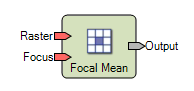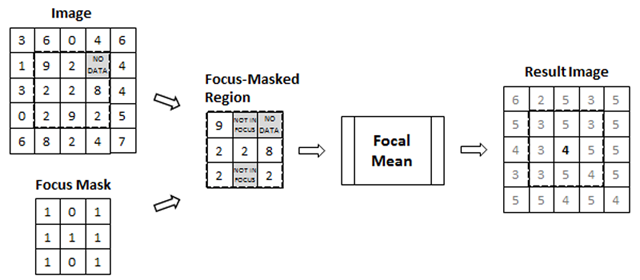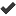# Focal Mean

## ERDAS IMAGINE Help

HGD_Variant
16.5.1
HGD_Product
ERDAS IMAGINE
HGD_Portfolio_Suite
Producer

Category: Focal

Function Type: NeighborhoodDescription

Focal Mean operates on a Raster dataset (usually thematic) using a neighborhood window. For each pixel in the image, it returns the mean of the pixels within the specified neighborhood. The output is a float Raster dataset with the same number of layers as the input dataset.

The Focus input is a user-defined binary Matrix that defines the neighborhood window. The size of the matrix defines the neighborhood search area. A True (nonzero) value in the matrix indicates the pixel underneath should be considered in the calculation; a False (zero) value indicates that the pixel should be ignored.

NoData values in the image are not considered in the calculations applied to their neighbors. NoData pixels can be replaced using the Replace NoData With operator.The following data types are supported:

 Input Output Binary not supported Integer Float Float Float Complex Complex Color not supported String not supported

Options for Ignoring Input Values

Three mutually exclusive options are available for ignoring values while running the focal function. If the combination of ignored values and NoData values result in no values for the focal function calculation, the FillWithNoData flag is used. If this flag is set to true, NoData will be output. If FillWithNoData is set to false, 0 will be used.

1. One or more values in the input dataset can be ignored by setting them on the Ignore Value input. A single value can be set as a scalar; multiple values are provided as a table.
2. One or more values to be used from the input dataset, with all others being excluded, can be set on the Use Value input. A single value can be set as a scalar; multiple values are provided as a table.
3. A binary lookup table can be set on the Use/Ignore Lookup Table input. With this option, when a value of N is encountered in the input dataset, it will be ignored unless the Nth entry in the lookup table is nonzero.

These options can be configured using the Focal dialog. Double-click on the operator in the Spatial Modeler Editor.

Options for Specifying Where to Apply the Function

Three mutually exclusive options are available to limit the application of the function to specific values or ranges in the input dataset. If the option specifies that the function is not to be applied at the value of a particular input pixel, the focal function is not calculated at that pixel, and the function returns the value of the input pixel. If the input pixel is NoData, the output pixel will be set to NoData. This means that if we are not applying at a particular value, the FillWithNoData flag will be ignored.

1. A value or table of values at which the function will not be applied can be set on the Don't Apply at Value input.
2. A value or table of values at which the function will exclusively be applied can be set on the Apply at Value input.
3. A binary lookup table can be set on the Apply Lookup Table input. With this option, when a value of N is encountered in the input dataset, the function will be applied only if the Nth entry in the lookup table is nonzero.

These options can be configured using the Focal dialog. Double-click on the operator in the Spatial Modeler Editor.

Connections

 Name Objects Supported Description Required Raster Raster Raster dataset on which to apply the focal function.Focus Matrix A matrix that is converted to binary and used as a mask to identify the focus region.Ignore Value Scalar, Table A single value or table of values to treat as NoData in the input dataset. Use Value Scalar, Table A single value or table of values that will be used from the input dataset, while all others are treated as NoData. Use/Ignore Lookup Table Table A table that is converted to binary and used as a lookup table for identifying valid values in the input dataset (which must be of integer type). A zero value in the lookup table indicates that the index value is to be treated as NoData. Don’t Apply At Value Scalar, Table A single value or table of values at which the focal function will not be applied. These values, when encountered in the input dataset, will be copied to the output dataset unchanged. Apply at Value Scalar, Table A single value or table of values at which to apply the focal function. All other values in the input dataset will be copied to the output dataset unchanged. Apply Lookup Table Table A table that is converted to binary and used as a lookup table for identifying values at which to apply the focal function. A zero value in the lookup table indicates that the index value is to be copied unchanged to the output dataset; a nonzero value indicates that the index value is to be processed through the focal function. FillWithNODATA Boolean A flag indicating whether to output NoData to the index pixel when the entire focus window contains either NoData or ignored values. If False, 0 will be used for this case. Output Raster The resulting raster dataset.

Syntax

Focal Mean ( <Raster>, <Focus> )

or

Focal Mean ( <Raster>, <Focus>, <use_option> )

or

Focal Mean ( <Raster>, <Focus>, <apply_option> )

or

Focal Mean ( <Raster>, <Focus>, <use_option>, <apply_option> )

or

FocalMean ( <Raster>, <Focus>[, IgnoreValue=<IgnoreValue>][, UseValue=<UseValue>][, UseI\-gnoreLookupTable=<UseIgnoreLookupTable>][, DontApplyatValue=<DontApplyatValue>][, Applyat\-Value=<ApplyatValue>][, ApplyLookupTable=<ApplyLookupTable>][, FillWithNODATA=<FillWithNODATA>] )

Notes

<use_option> is one of the following:

`	IGNORE_VALUE <value>	IGNORE_VALUE { <value1> , <value2> , <value3> , ... }	IGNORE_VALUE <table>	USE_VALUE <value>	USE_VALUE { <value1> , <value2> , <value3> , ... }	USE_VALUE <table>	USE_LOOKUP_TABLE <binary_table>`

<apply_option> is one of the following:

`	NO_APPLY_AT_VALUE <value>	NO_APPLY_AT_VALUE { <value1> , <value2> , <value3> , ... }	NO_APPLY_AT_VALUE <table>	APPLY_AT_VALUE <value>	APPLY_AT_VALUE { <value1> , <value2> , <value3> , ... }	APPLY_AT_VALUE <table>	APPLY_LOOKUP_TABLE <binary_table>`

<value>, <value1>, <value2> and so on are Scalar expressions. <table> and <binary_table> are Tables.

A <use_option> specifies which values are to be used in computing the focal function. IGNORE_VALUE specifies that the values in <value> or <table> will not be used. USE_VALUE specifies that only the values in <value> or <table> are used, and all other values are ignored. USE_LOOKUP_TABLE specifies that its <binary_table> will be cast to binary and used as a binary lookup table to determine whether or not a value is used: True indicates to use the value, False to ignore it. For more information about how the ignore/use values are treated in the focal operation, see Options for Ignoring Input Values, above.

An <apply_option> specifies whether or not the focal function is calculated for a particular input pixel value. NO_APPLY_AT_VALUE specifies that the function will not be applied at values in <value> or <table>. APPLY_AT_VALUE specifies that the function is applied only at the values in <value> or <table>, and at no other values. APPLY_LOOKUP_TABLE specifies that its <binary_table> will be cast to binary and used as a binary lookup table to determine whether or not to apply the function at a value: True indicates to apply at the value, False indicates not to apply at the value. For more information about how the apply values are treated in the focal operation, see Options for Specifying Where to Apply the Function, above.